SLUSBZ9C August   2015  – September 2016

PRODUCTION DATA.

1. Features
2. Applications
3. Description
4. Revision History
5. Description (continued)
6. Device Comparison Table
7. Pin Configuration and Functions
8. Specifications
9. Detailed Description
10. 10Application and Implementation
1. 10.1 Application Information
2. 10.2 Typical Application
1. 10.2.1 Design Requirements
2. 10.2.2 Detailed Design Procedure
3. 10.2.3 Application Performance Curves
11. 11Power Supply Recommendations
12. 12Layout
13. 13Device and Documentation Support
14. 14Mechanical, Packaging, and Orderable Information

• YFP|25

## 10 Application and Implementation

NOTE

Information in the following applications sections is not part of the TI component specification, and TI does not warrant its accuracy or completeness. TI's customers are responsible for determining suitability of components for their purposes. Customers should validate and test their design implementation to confirm system functionality.

### 10.1 Application Information

A typical design is shown in Figure 35. This design uses the BQ25120 with external resistors for ILIM, IPRETERM, and ISET. These are not needed if these values are set with a host controller through I2C commands. This design also shows the TS resistors, which is also optional.

When powering up in default mode the battery voltage is the default for the part (4.2 V), the SYS output is the default (1.8 V). External resistors set the charge current to 40 mA, the termination current to 10% (4 mA), and the input current limit to 100 mA. If the I2C interface is used the part goes to the internal default settings until changed by the host.

### 10.2 Typical Application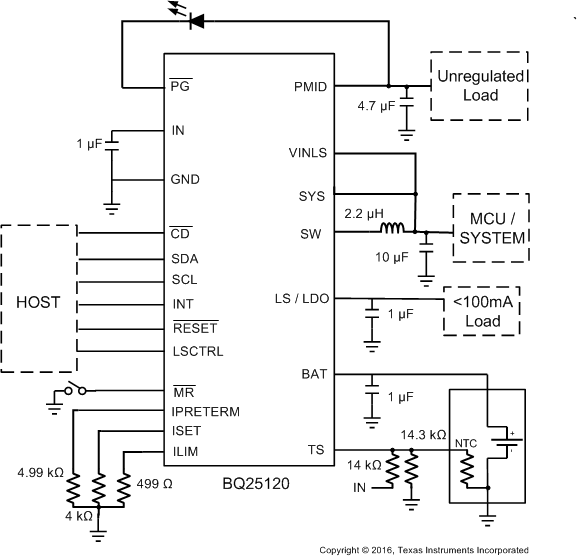Figure 35. Typical Application Circuit

#### 10.2.1 Design Requirements

This application is for a low power system that has varying loads from less than 10 mA up to 300 mA. It must work with a valid adaptor or USB power input. Below are some of the key components that are needed in normal operation. For this example, the fast charge current is 50 mA, input current limit is 400 mA and the pre-charge and termination current is 10% of the fast charge current.

• Supply voltage = 3.4 V to 20 V
• Fast charge current is default to 10 mA with ISET pin shorted to ground. To program the fast charge current, connect an external resistor from ISET to ground.
• Input current limit is default to 100 mA with ILIM pin shorted to ground. To program the input current limit, connect an external resistor from ILIM to ground.
• Termination current threshold is default to 2 mA with IPRETERM pin shorted to ground. To program the input current limit, connect an external resistor from IPRETERM to ground.
• A 2.2-µH inductor is needed between SW pin and SYS pin for PWM output.
• TS- Battery temperature sense needs a NTC connected on TS pin.

#### 10.2.2 Detailed Design Procedure

See Figure 35 for an example of the application diagram.

#### 10.2.2.1 Default Settings

• • Connect ISET, ILIM and IPRETERM pins to ground to program fast charge current to 10mA, input current limit to 100mA and pre-charge/termination current to 2 mA.
• BAT_UVLO = 3 V.
• VSYS = 1.8 V
• LS/LDO is LS
• VBREG = 4.2 V
• VIN_DPM is enabled and VIN_DPM Threshold = 4.6 V.
• Safety Timer = 3 hr
• If the function is not needed, connect TS to the center tab of the resistor divider between VIN and the ground. (pull up resistor = 14 kΩ, pull down resistor = 14.3 kΩ)

#### 10.2.2.2 Choose the Correct Inductance and Capacitance

Refer to the Buck (PWM) Output section for the detailed procedure to determine the optimal inductance and capacitance for the buck output.

#### 10.2.2.3.1 Program the Fast Charge Current (ISET)

Equation 10. RISET = KISET/ICHG

KISET = 200 AΩ from the Specifications table

Equation 11. RISET = 200 AΩ / 0.05A = 4 kΩ

Select the closest standard value, which in this case is 4.99 kΩ. Connect this resistor between ISET pin and GND.

#### 10.2.2.3.2 Program the Input Current Limit (ILIM)

Equation 12. RILIM = KILIM/II_MAX

KILIM = 200 AΩ from the Specifications table

Equation 13. RILIM = 200 AΩ / 0.4A = 500 Ω

Select the closest standard value, which in this case is 499 Ω. Connect this resistor between ILIM pin and GND.

#### 10.2.2.3.3 Program the Pre-charge/termination Threshold (IPRETERM)

According to Table 3, the RIPRETERM is 4990 Ω for 10% termination threshold. Therefore, connect a 4.99 kΩ resistor between IPRETERM pin and GND.

#### 10.2.2.3.4 TS Resistors (TS)

The voltage at TS is monitored to determine that the battery is at a safe temperature during charging. This device uses JEITA temperature profile which has four temperature thresholds. Refer to Specifications for the detailed thresholds number.

The TS circuit is shown in Figure 16. The resistor values can be calculated using Equation 1 and Equation 2.

#### 10.2.3.1 Charger Curves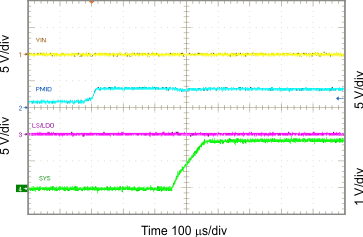Figure 36. Battery Connected to V(BAT)Figure 38. Entering DPPM Mode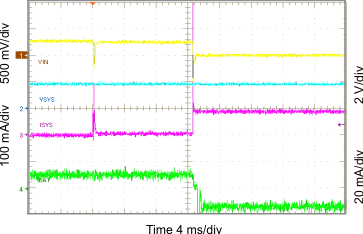Figure 40. Entering Battery Supplement Mode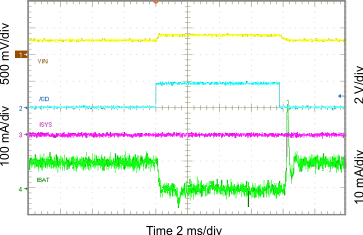Figure 42. Charger On/Off Using CD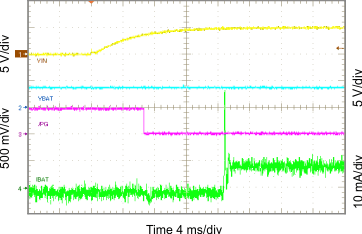Figure 37. Power Supply Connected to VIN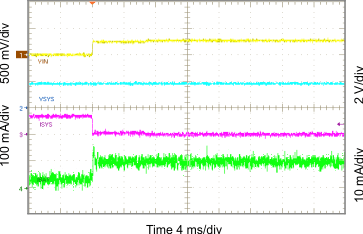Figure 39. Exiting DPPM Mode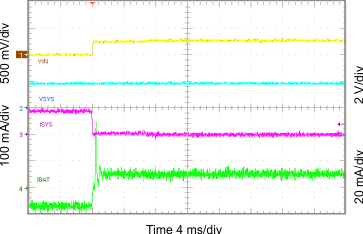Figure 41. Exiting Battery Supplement Mode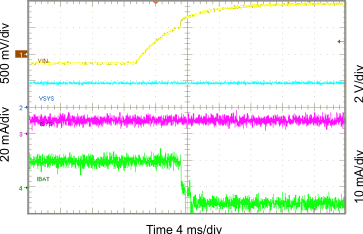Figure 43. OVP Fault

#### 10.2.3.2 SYS Output Curves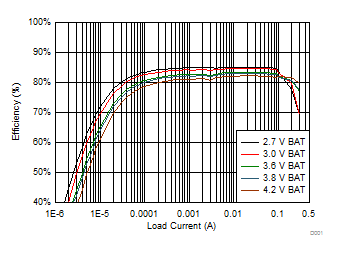TA = 25°C VSYS = 1.2 V
Figure 44. 1.2 VSYS System Efficiency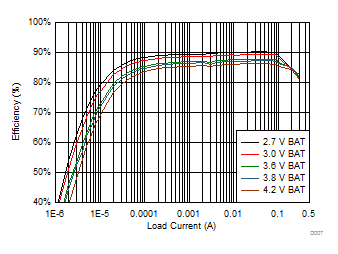TA = 25°C VSYS = 1.8 V
Figure 46. 1.8 VSYS System Efficiency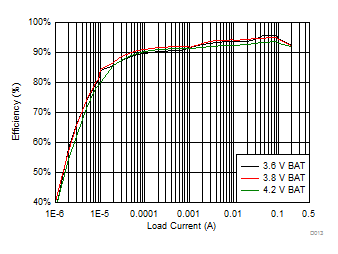TA = 25°C VSYS = 3.3 V
Figure 48. 3.3 VSYS System EfficiencyTA = 25°C VSYS = 1.5 V
Figure 50. 1.5 VSYS Load Regulation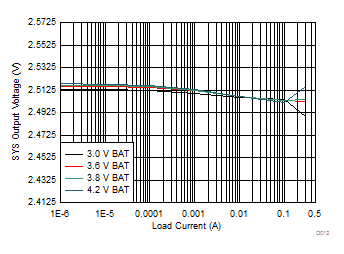TA = 25°C VSYS = 2.5 V
Figure 52. 2.5 VSYS Load Regulation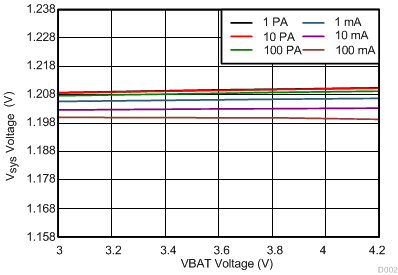TA = 25°C VSYS = 1.2 V
Figure 54. 1.2 VSYS Line Regulation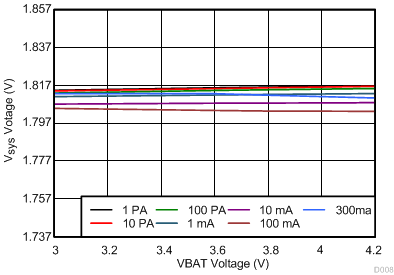TA = 25°C VSYS = 1.8 V
Figure 56. 1.8 VSYS Line Regulation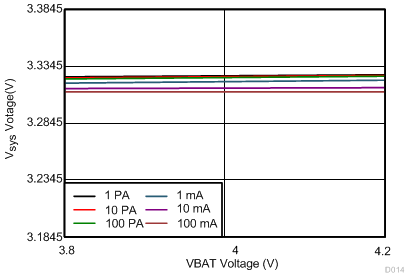TA = 25°C VSYS = 3.3 V
Figure 58. 3.3 VSYS Line Regulation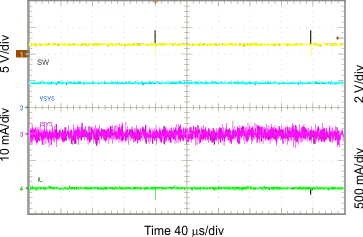Figure 60. Light Load Operation Showing SW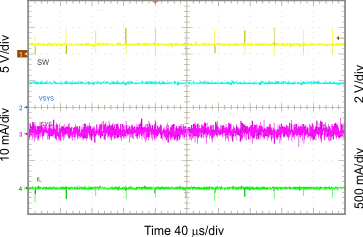Figure 62. Light Load Operation Showing SWFigure 64. Light Load Operation Showing SW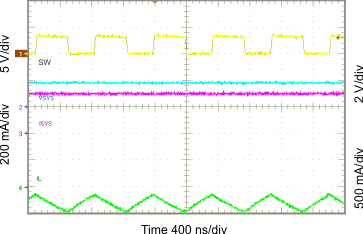Figure 66. Light Load Operation Showing SW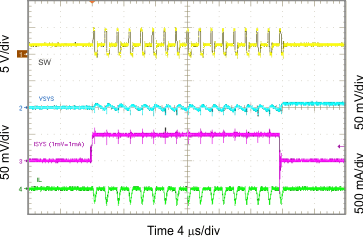VSYS = 1.8 V
Figure 68. 1.8 VSYS Load Transient, 0 to 50 mA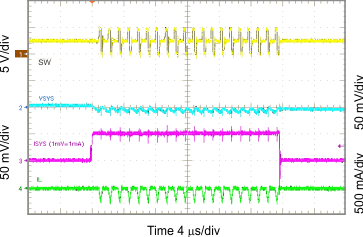VSYS = 2.5 V
Figure 70. 2.5 VSYS Load Transient, 0 to 50 mA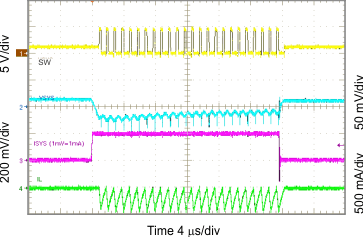VSYS = 1.2 V
Figure 72. 1.2 VSYS Load Transient, 0 to 200 mA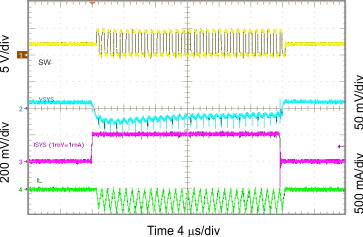VSYS = 2.1 V
Figure 74. 2.1 VSYS Load Transient, 0 to 200 mA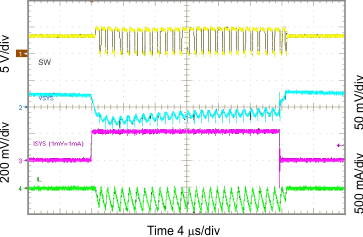VSYS = 3.3 V
Figure 76. 3.3 VSYS Load Transient, 0 to 200 mA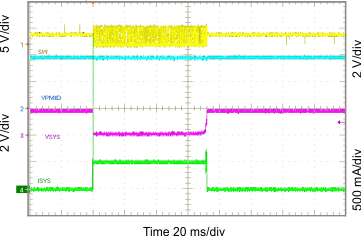Figure 78. Short Circuit and Recovery for SYS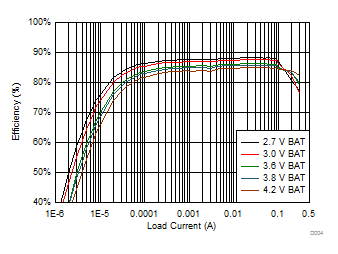TA = 25°C VSYS = 1.5 V
Figure 45. 1.5 VSYS System Efficiency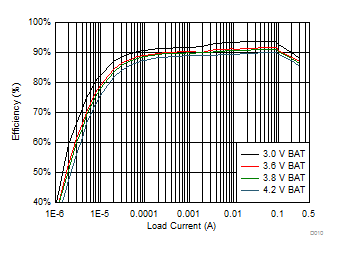TA = 25°C VSYS = 2.5 V
Figure 47. 2.5 VSYS System Efficiency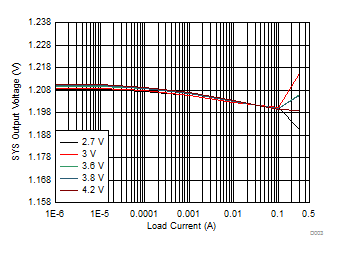TA = 25°C VSYS = 1.2 V
Figure 49. 1.2 VSYS Load Regulation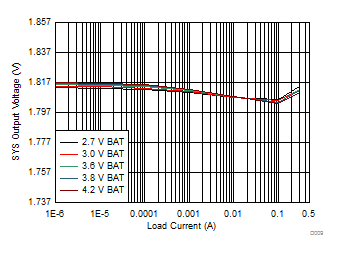TA = 25°C VSYS = 1.8 V
Figure 51. 1.8 VSYS Load Regulation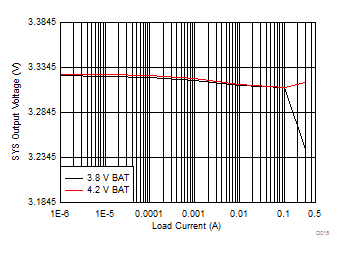TA = 25°C VSYS = 3.3 V
Figure 53. 3.3 VSYS Load Regulation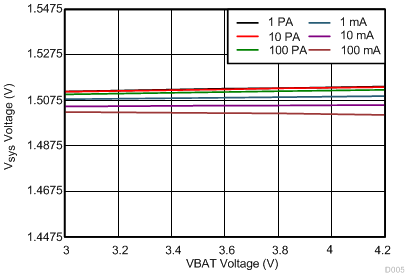TA = 25°C VSYS = 1.5 V
Figure 55. 1.5 VSYS Line Regulation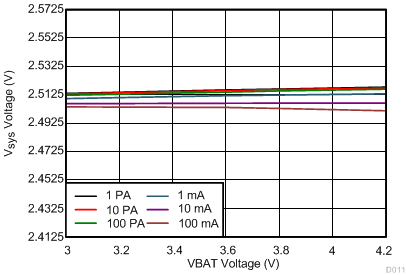TA = 25°C VSYS = 2.1 V
Figure 57. 2.1 VSYS Line Regulation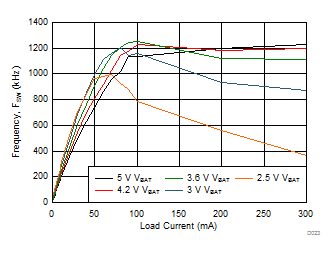Figure 59. 1.8 VSYS Switching Frequency vs Load Current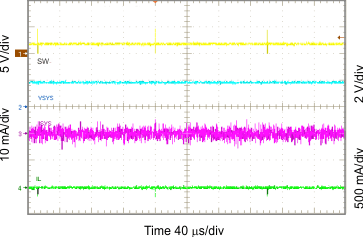Figure 61. Light Load Operation Showing SW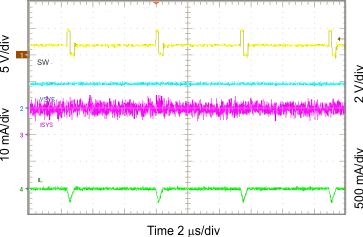Figure 63. Light Load Operation Showing SW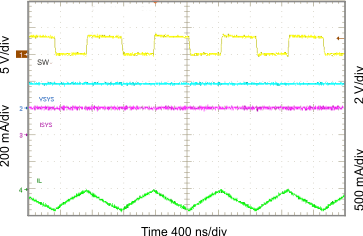Figure 65. Light Load Operation Showing SW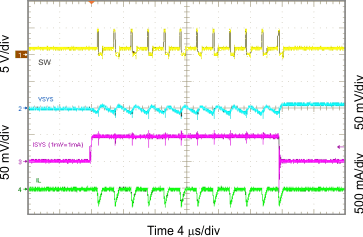VSYS = 1.2 V
Figure 67. 1.2 VSYS Load Transient, 0 to 50 mA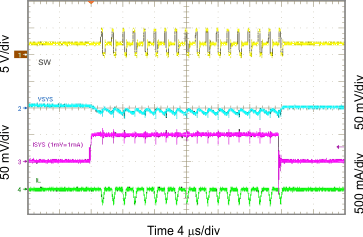VSYS = 2.1 V
Figure 69. 2.1 VSYS Load Transient, 0 to 50 mA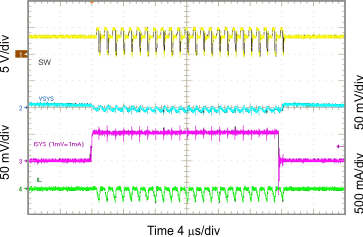VSYS = 3.3 V
Figure 71. 3.3 VSYS Load Transient, 0 to 50 mA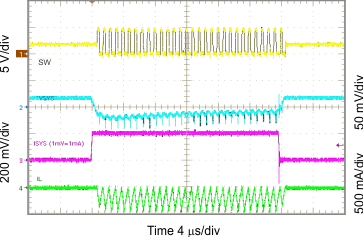VSYS = 1.8 V
Figure 73. 1.8 VSYS Load Transient, 0 to 200 mA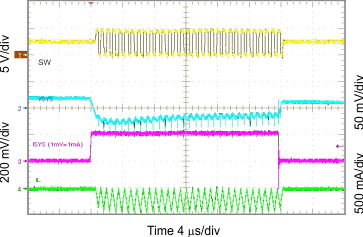VSYS = 2.5 V
Figure 75. 2.5 VSYS Load Transient, 0 to 200 mA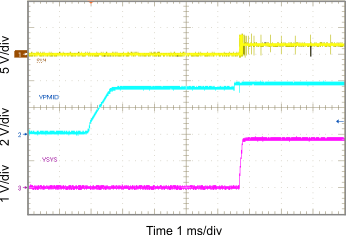Figure 77. Startup Showing SS on SYS in PWM Mode

#### 10.2.3.3 Load Switch and LDO Curves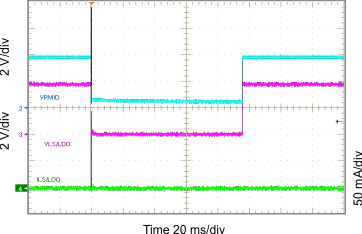Figure 79. Short Circuit and Recovery for LS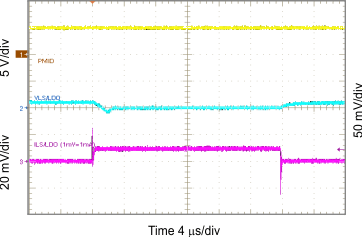VSLSDO = 0.8 V
Figure 81. 0.8 VLSLDO Load Transient, 0 to 10 mA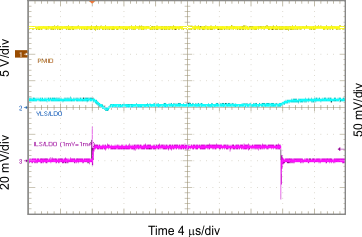VSLSDO = 1.8 V
Figure 83. 1.8 VLSLDO Load Transient, 0 to 10 mA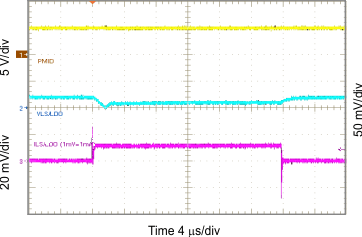VSLSDO = 3.3 V
Figure 85. 3.3 VLSLDO Load Transient, 0 to 10 mA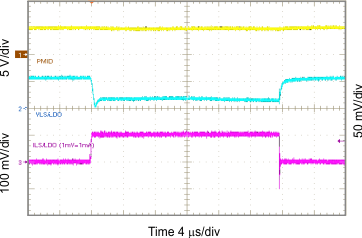VSLSDO = 1.2 V
Figure 87. 1.2 VLSLDO Load Transient, 0 to 100 mA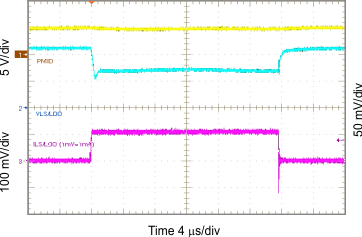VSLSDO = 2.5 V
Figure 89. 2.5 VLSLDO Load Transient, 0 to 100 mA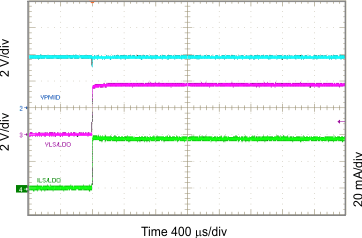Figure 80. Startup Showing SS on LS/LDO Output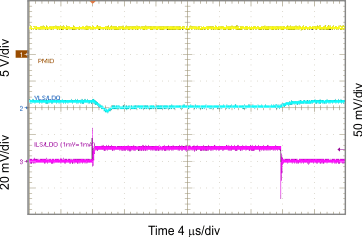VSLSDO = 1.2 V
Figure 82. 1.2 VLSLDO Load Transient, 0 to 10 mA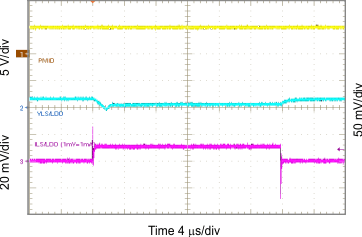VSLSDO = 2.5 V
Figure 84. 2.5 VLSLDO Load Transient, 0 to 10 mA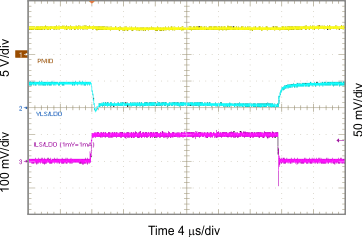VSLSDO = 0.8 V
Figure 86. 0.8 VLSLDO Load Transient, 0 to 100 mA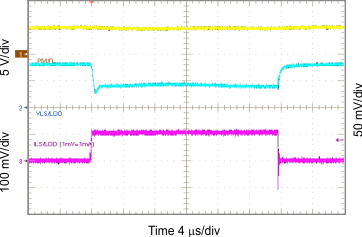VSLSDO = 1.8 V
Figure 88. 1.8 VLSLDO Load Transient, 0 to 100 mA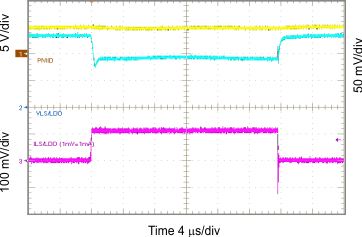VSLSDO = 3.3 V
Figure 90. 3.3 VLSLDO Load Transient, 0 to 100 mA

#### 10.2.3.4 LS/LDO Output Curves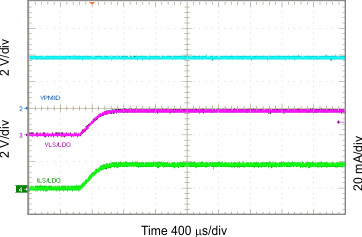Figure 91. Startup Showing SS on LS/LDO in LDO Mode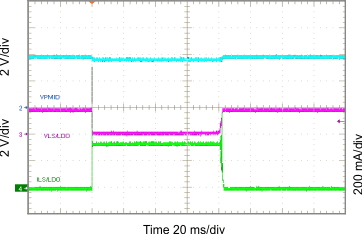Figure 92. Short Circuit and Recovery for LDO

#### 10.2.3.5 Timing Waveforms Curves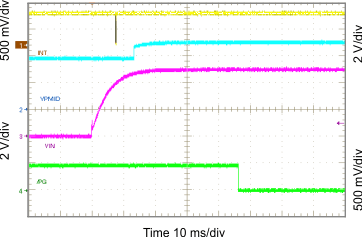Figure 93. Show PG and INT Timing (VIN Insertion)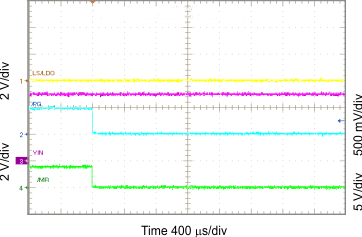Figure 95. PG Functions as Shifted MR Output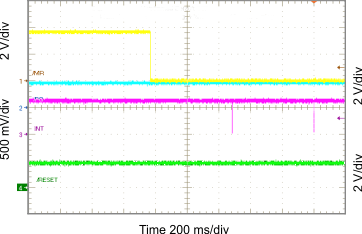Wake1 = 500 ms Wake2 = 1 s
Figure 97. Show MR Timing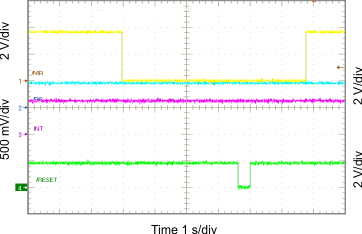RESET = 4 s
Figure 99. RESET Timing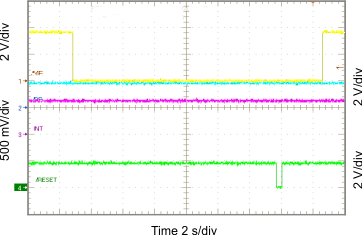RESET = 14 s
Figure 101. RESET Timing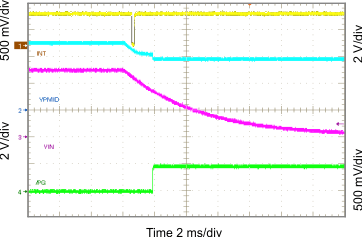Figure 94. Show PG and INT Timing (VIN Removal)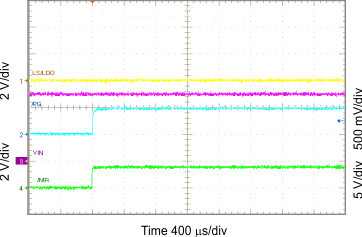Figure 96. PG Functions as Shifted MR Output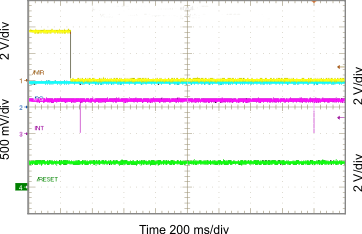Wake1 = 50 ms Wake2 = 1.5 s
Figure 98. Show MR Timing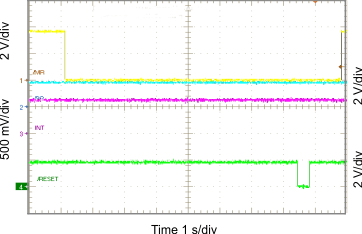RESET = 8 s
Figure 100. RESET TimingFigure 102. RESET Timing and Enter Ship Mode